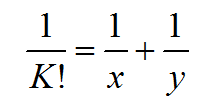Problem Statement
Given a positive integer K, find the number of distinct positive integer solutions of the following equation.Input Specifications
The first line of input contains T, the number of test cases.
Each test case consists of a positive integer K in a separate line.

Output Specifications
For each test case, print the answer followed by a new line.

Constraints
• 1 <= T <= 1000

• 1 <= K <= 10000

Sample Input
``` 3 1 2 3 ```

Sample Output
``` 1 3 9 ```

Explanation:
Case #1: The only possible solution is (2, 2)
Case #2: The possible solutions are (4, 4), (3, 6) and (6, 3)

Problem Setter: Rohan Laishram

Languages: C#,JavaScript,Pascal,Perl,PHP,Python,Ruby

Time Limit: 6 Second(s)
Score: 100 Point(s)
Input File Limit: 50000 Bytes

Mode Judge

RankNameScore
1xyz0
2Ams0
3TIP0
4team420
5xyzz0
6asdasdasd0
7abcd0
8khankhan0
9Gabriel0
10gigel0